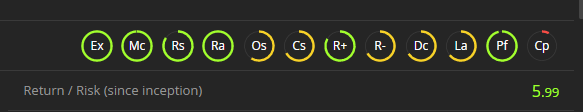1. Darwinex Docs
2. World of Darwinex
3. Darwinex Metrics and Graphs

# Return/Risk Ratio

## The Return/Risk ratio gives an estimate of how much return is obtained per ''unit of risk''.

The Return/Risk ratio gives an estimate of how much return is obtained per ''unit of risk''.It enables you to compare strategies in regards to the risk they can handle.

It is calculated by carrying out random strategy simulations which trade the same assets as the average strategy, and comparing them.

To have an idea of how good a strategy is:

• Above 1, the strategy beats 68% of random strategies, it is good
• Above 1.65, the strategy beats 95%, it is very good
• Above 2, the strategy beats 99%, it is impressive